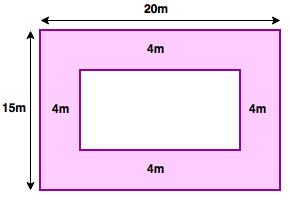# Area and Perimeter - Aptitude Questions and Answers

## Area and Perimeter Questions and Answers

Learn and practice the chapter "Area and Perimeter" with these solved Aptitude Questions and Answers. Each question in the topic is accompanied by a clear and easy explanation, diagrams, formulae, shortcuts and tricks that help in understanding the concept.

## Use of Area and Perimeter Questions

The questions and examples given in this section will be useful to all the freshers, college students and engineering students preparing for placement tests or any competitive exam like MBA, CAT, MAT, SNAP, MHCET, XAT, NMAT, GATE, Bank exams - IBPS, SBI, RBI, RRB, SSB, SSC, UPSC etc.

Practice with this online test to crack your placements and entrance tests!
1. A triangular area has to be painted with neon paint. The cost of painting is Rs 80 per sq km.
The sides of the area are 8 km, 10 km and 6 km. Find the cost that will be incurred on painting it.

a. Rs. 1920
b. Rs. 2560
c. Rs. 3840
d. Rs. 38400

Explanation:

Tip:
Sides of triangle = a, b and c
Area = 2s(s-a)(s-b)(s-c)
 Here value of s = a+b+c 2

 s = a+b+c = 8+6+10 = 12 2 2
Area = 2s(s-a)(s-b)(s-c) = 212(12-6)(12-10)(12-8)
∴ Area = 212 x 6 x 2 x 4 = 24 sq.km
Cost = Rate x Area = 80 x 24 = Rs. 1920

2. Three triangles, △LMN, △PQR and △CDE are formed in such a way that the inside triangle is formed by joining the midpoints of the sides of outside triangle. △LMN is the outermost triangle while △CDE is the innermost triangle. The length of the sides of inner most triangle are CD = 3, DE = 8 and CE = 4. Find the perimeter of the outermost triangle.

a. 30
b. 60
c. 72
d. 90

Explanation:

We can draw the triangles to understand the sum betterTip:
When you join midpoints of sides of a triangle and form a smaller triangle, then
Bigger △'s Area = 2 x Area of Smaller △
Bigger △'s Perimeter = 2 x Perimeter of Smaller △

∴ Perimeter of △PQR = 2 x Perimeter of △CDE = 2(3+8+4) = 30
Perimeter of △LMN = 2 x Perimeter of △PQR = 2 x 30 = 60

3. The length and breadth of a rectangular piece of fabric are in the ratio 7:4. The length of the fabric is 15 m more than that of the breadth. Find the area that this fabric would cover.

a. 450 sq.m.
b. 700 sq.m.
c. 850 sq.m.
d. 900 sq.m.

Explanation:

Let the common factor be K
∴ Length = 7K and Breadth = 4K
Also, 4K+15m = 7K
∴ K = 5
∴ Area of Rectangular ground = Length x Breadth = 7K x 4K = 35 x 20 = 700 sq.m.

4. A rectangular piece of paper has an area 840 sq. cm. The length of the side is 48 cm. The
 rectangle is stretched such that its area becomes 2 6 times original rectangle. The only dimension 7
that changes is the breadth of the rectangle. Find the perimeter of the new rectangle.

a. 1.69 m
b. 1.85 m
c. 1.96 m
d. 2.25 m

Explanation:

 New area = 840 x 2 6 = 840 x 20 = 2400 sq.cm 7 7
Area of Rectangle = length x breadth
 New Breadth = New Area = 2400 = 50 cm Length 48
Perimeter of new margin = 2(length + new breadth) = 2(48 + 50) = 196cm = 1.96m

5. Sheetal has a rectangular piece of cloth with dimensions 20 m and 15 m. She wants to paint a border of breadth 4 m inside the four sides of the rectangle. The paint would cost her Rs 6 per sq m. Find the cost of painting the complete border.

a. Rs. 1083
b. Rs. 1296
c. Rs. 1500
d. Rs. 1548